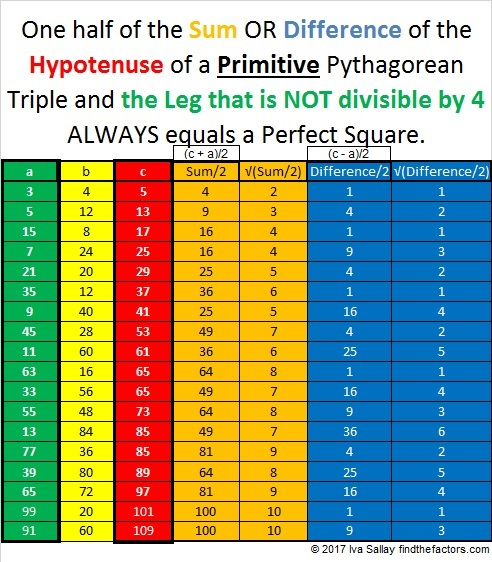# 857 Why do Primitive Pythagorean Triples Do That?

29² + 4² = 857, so 857 is the hypotenuse of a Pythagorean triple that just happens to be primitive:

• 232-825-857 which was calculated from 2(29)(4), 29² – 4², 29² + 4².

Now, one of the legs of a primitive Pythagorean triple will be divisible by 4. In this case that number is 232. As I indicated when I wrote 821 Why Do Primitive Pythagorean Triples Do That? The sum of the number divisible by 4 in a primitive Pythagorean triple and the hypotenuse always equals a perfect square. So 232 + 857 = 1089 = 33².

This week I noticed something about the OTHER leg that might be even more amazing! The other leg in the triple, 825, is not divisible by 4, but get this: One half of the sum of a primitive’s hypotenuse and that leg that is NOT divisible by 4 also equals a square number: For example, (857 + 825)/2 = 1681/2 = 841 = 29².

But not only that, one half of the DIFFERENCE of a primitive’s hypotenuse and that leg that is NOT divisible by 4 equals a perfect square, too: (857 – 825)/2 = 32/2 = 16 = 4².

Now 29² and 4² look a little familiar to me today. Hmm… We’ve seen those two numbers together earlier in this post!

It seems that for Primitive Pythagorean Triples, what goes around comes around!

Check out the sums and difference of these other primitive Pythagorean triples:Go ahead, try it with any other primitive triple on this list or otherwise!

If we let s = √(Sum/2) and let d = √(Difference/2), then we can rewrite some Pythagorean triple like this:

• s² – d², 2sd, s² + d², so s² and d² are REALLY significant perfect squares!

Besides being the hypotenuse of a primitive Pythagorean triple, here are some other facts about the number 857:

857 is the sum of three consecutive prime numbers: 281 + 283 + 293.

857 is also the sum of the nineteen prime numbers from 11 to 83.

• 857 is a prime number.
• Prime factorization: 857 is prime.
• The exponent of prime number 857 is 1. Adding 1 to that exponent we get (1 + 1) = 2. Therefore 857 has exactly 2 factors.
• Factors of 857: 1, 857
• Factor pairs: 857 = 1 × 857
• 857 has no square factors that allow its square root to be simplified. √857 ≈ 29.27456

How do we know that 857 is a prime number? If 857 were not a prime number, then it would be divisible by at least one prime number less than or equal to √857 ≈ 29.3. Since 857 cannot be divided evenly by 2, 3, 5, 7, 11, 13, 17, 19, 23, or 29, we know that 857 is a prime number.

Here’s another way we know that 857 is a prime number: Since its last two digits divided by 4 leave a remainder of 1, and 29² + 4² = 857 with 29 and 4 having no common prime factors, 857 will be prime unless it is divisible by a prime number Pythagorean triple hypotenuse less than or equal to √857 ≈ 29.3. Since 853 is not divisible by 5, 13, 17, or 29, we know that 857 is a prime number.